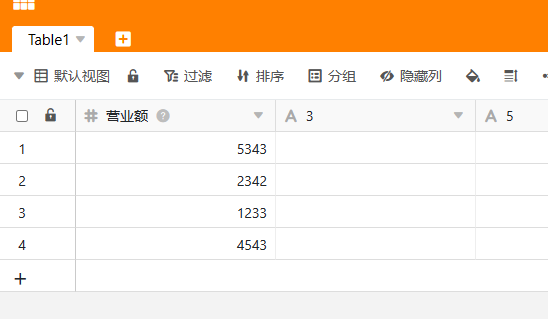# Python 脚本中怎么获取行的ID``````rows = base.list_rows('Table1')
row_id_1 = rows.get("_id")
row_id_2 = rows.get("_id")
``````

``````row_1 = base.query("select _id from Table1 where No=1")
row_1_id = row_1.get("_id")
``````
1 个赞